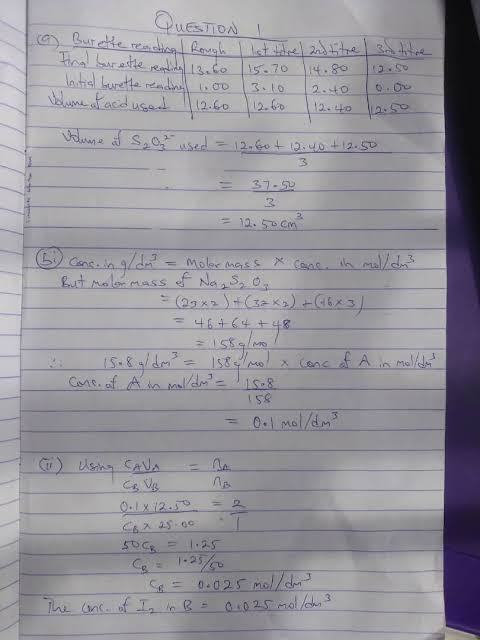-->

# wace 2022 CHEMISTRY practical QUESTIONS AND Answers

## CHEMISTRY QUESTIONS AND Answers

### 14th JUNE 2022

chemestry

QUESTIONS PRACTICAL 1

(1a)
Volume of pipette used, VA = 25.0cm³

[TABULATE]

Burette Reading |  Rough titre | 1st titre | 2nd titre |

Final Burette reading (cm³) |  25.60 | 32.80 | 26.40

Initial Burette reading (cm³) | 1.20 | 8.70 | 2.30

Volume of Na₂S₂O₃ used | 24.40 | 24.10 | 24.10

Average burette reading = (24.10 + 24.10)/2
Average volume of Na₂S₂O₃ used = 24.10cm³
VB = 24.10cm³

(1bi)
Given: CB = 0.1mol/dm³
VB = 24.10cm³
VA = 25.0cm³
CA =?
From equation nA/nB = 1/2
.: Using (CABA)/CBVB = (nA)/nB

(CA x 25)/(0.1x24.10) = 1/2
CA = (0.1x24.10)/(25x2)
CA = 0.0482mol/dm³
.: Concentration of iodine in A = 0.0482mol/dm³

(1bii)
Mass in gramms of iodine in 1dm³ of A = Molarity × Molar mass of iodine
= 0.0482×127(2)
= 12.2428g/dm³

TAKE NOTE

FOR QUESTION ONE

Use your school's endpoint and use it wherever you see 24.10cm³....and calculator with it

Use the answer in 1b(i) to solve in 1b(Ii)======================================================
======================================================
QUESTIONS PRACTICAL 2

(2a)
[TABULATE]

=TEST=
C + Distilled water

=OBSERVATION=
It dissolves completely to give a light green solution.

=INFERENCE=
Soluble salt.

(2ai)
=TEST=
Solution C + NaOH in drops and in excess + Heat gently

=OBSERVATION=
A dirty green precipitate is formed which remains insoluble in excess.
Effervescence occurs in which a colourless gas with a pungent smell which turns red litmus blue is given off.

=INFERENCE=
Fe²⁺ is present.
NH₃ gas form.
NH₄⁺ is present.

(2aii)
=TEST=
Solution + BaCl₂ + dilute HCL in excess

=OBSERVATION=
A white precipitate is formed.
The white precipitate remains insoluble and gives a white dense.

=INFERENCE=
SO₄²⁻, CO₃²⁻, SO₃²⁻, is present.
SO₄²⁻ confirmed.

(2b)
Cations → Fe²⁺ and NH₄⁺
Anions → SO₄²⁻

=====================================================
======================================================
QUESTIONS PRACTICAL 3

(3a)
Sulphur Dioxide Solution Turns Acidified Potassium Chromate Solution green Where As carbon dioxide Shows no Change

(3bi)
(I) H2 ; downward displacement of water
(II) NH3; downward displacement of air
(III) HCL; upward displacement of air

(3bii)
(I) Hydrogen gas(H2) is collected by the downward displacement of water because It is insoluble in water and It form an explosive mixture with air.

(II) Ammonia gas(NH3) is collected by downward displacement of air because it is lighter than air

(III) HCl gas is collected by upward displacement of air because it is 1.28 times heavier than air.

(3c)
(i) Distillation
(ii) Filtration followed by evaporation to dryness

(3d)This is because KCl react with NaHCO₃ to form two salts

GOOD LUCK